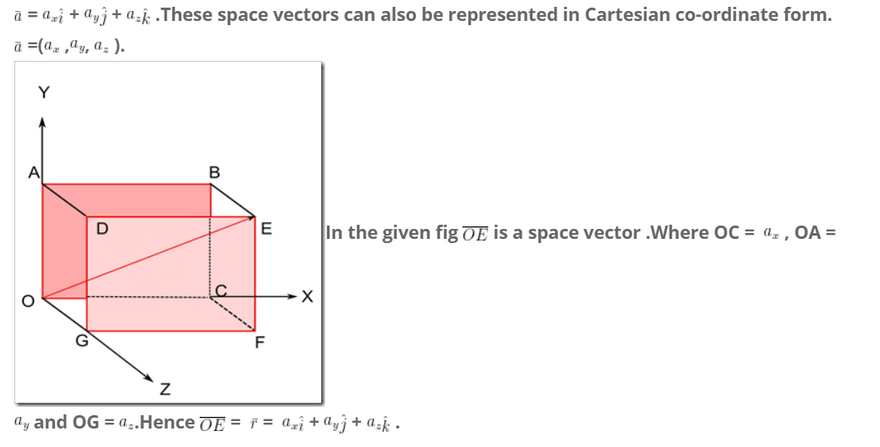# Vectors

Physical quantities are classified in to two categories i) Scalar quantities ii)Vector quantities

1. Scalar quantities or Scalars :The physical quantities which have magnitude but no direction are called scalar quantities or scalars. Ex: Length, mass, time, area, volume, speed, energy, work ,temperature etc. 2.Vector quantities or Vectors: The physical quantities which posses magnitude as well as direction are called Vector quantities or Vectors. Ex: Displacement, velocity, acceleration, momentum, force etc Geometrical representation of vectors: A vector is geometrically represented by a directed line segment. The length of the directed line segment is called magnitude of the vector and the direction of the line segment represents the direction of the vector quantity.Ex: If a body starts from a point O after traveling for certain time it reaches its destination point A. Then the displacement of the body is represented by the directed line segment OA . The Length of the straight line OA represents the magnitude of the displacement of the body, and the direction of the line segment from O towards A represents the direction of displacement of the body. Vector overline{AB} is read as AB bar. The magnitude of overline{AB} is written as mid over line{AB}mid and read as modulus of overline{AB}.

Types of Vectors:
1. Co-initial vectors: All those vectors whose initial points are same, such vectors are called co initial vectors.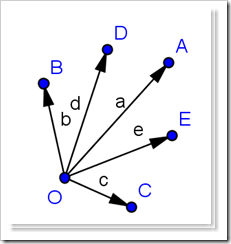Ex: OA,OB,OC, OD ,OE all these vectors have initial point O. Hence these are co-initial vectors.

2. Co-terminal vectors: All those vectors whose terminal (end) points are same, such vectors are called co terminal vectors.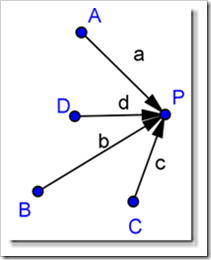Ex: The terminal point of all these vectors overline{AP},overline{BP},overline{CP} ,overline{DP} is Same point P. Hence, these vectors are called co-terminal vectors.

3. Coplanar Vectors: The vectors which lie in the same plane are called Co-planar Vectors.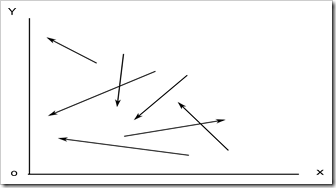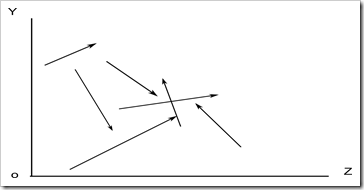Ex: a) All the vectors which lie in XY plane are called coplanar irrespective of their magnitudes
and directions. b) all the vectors which lie in YZ plane are coplanar with respect to one another.

4. Null vectors: Any vector which has direction but no magnitude is called a null vector. For a null
vector its initial point and the terminal point will be same .
Ex: overline{AA} ,overline{BB} . overline{OO} ………………. Are null vectors.

5. Unit Vector: Any vector of unit magnitude is called unit vector. If bar{a} is a vector, it’s unit vector is denoted by hat{a}.This (hat{a}) is read as a cap.
If you divide a vector with its magnitude we get the unit vector of that vector.
Therefore unit vector hat{a}= frac{bar{a}}{ mid bar{a} mid}
where mid bar{a} mid is called the magnitude of bar{a} vector.
Every vector will have its own unit vector.Unit vector of any vector will be of unit magnitude irrespective of the magnitude of the original vector.
If bar{b} is a vector its unit vector will be hat{b} ,the direction of bar{b} and hat{b} will be same.Similarly if bar{c} is a vector its unit vector will be hat{c} ,the direction of bar{c} and hat{c} will be same.
Three mutually perpendicular axes OX,OY AND OZ form the Cartesian co-ordinate system.The unit vectors along X,Y and Z axis are represented by hat{i} ,hat{j} and hat{k} respectively.
6. Like vectors: All the vectors acting in the same direction are called like vectors.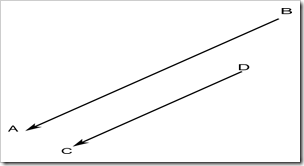The given vectors overline{AB} and overline{DC}
are acting in the same direction ,hence overline{AB} and overline{DC} are like vectors.
Note: Like vectors will be always i)parallel to one another ii) will be acting in the same direction , but iii)not necessarily be of the different magnitudes.

7. Unlike Vectors: Any two vectors parallel to one another and acting in opposite directions are called unlike vectors.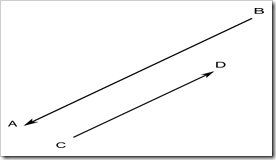The given vectors overline{AB} and overline{CD}are acting in the opposite directions , hence overline{AB} and overline{CD} are unlike vectors.
Note: Unlike vectors will be always i)parallel to one another ii) will be acting in the opposite directions , but iii)not necessarily be of the different magnitudes.

8. Negative Vector: If overline{AB}= bar{a} is a vector,then overline{DC} = –bar{a} will be the negative vector of bar{a}.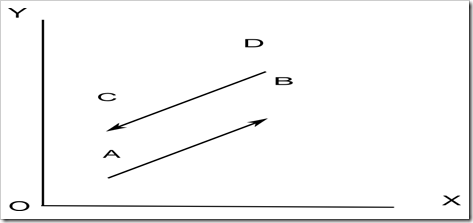1. The original vector bar{a} and it’s negative vector –bar{a} will be of same magnitude i.e mid bar{a} mid = mid overline{-a} mid
2. They will be opposite to one another. iii) every vector will have a negative vector.

9. Axial Vectors or (One dimensional Vectors): The vectors acting along X,Y or Z axes ( or) vectors parallel to any one of the Axis are called axial vectors (or) one dimensional vectors.
9(i). Vectors parallel to X axis : In the Fig(1) all the vectors overline{AB} = bar{a} ,overline{CD} = bar{b} and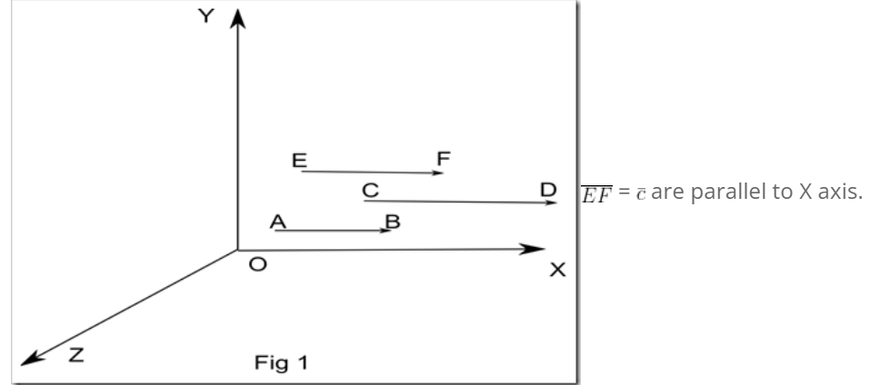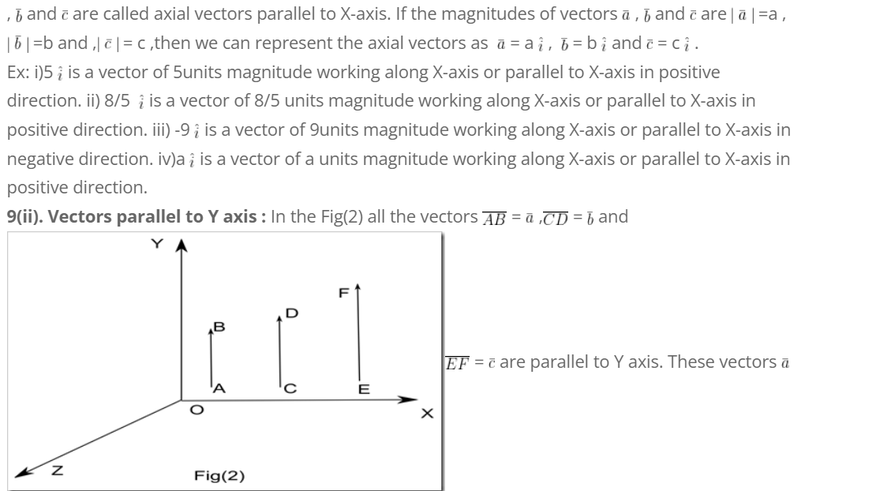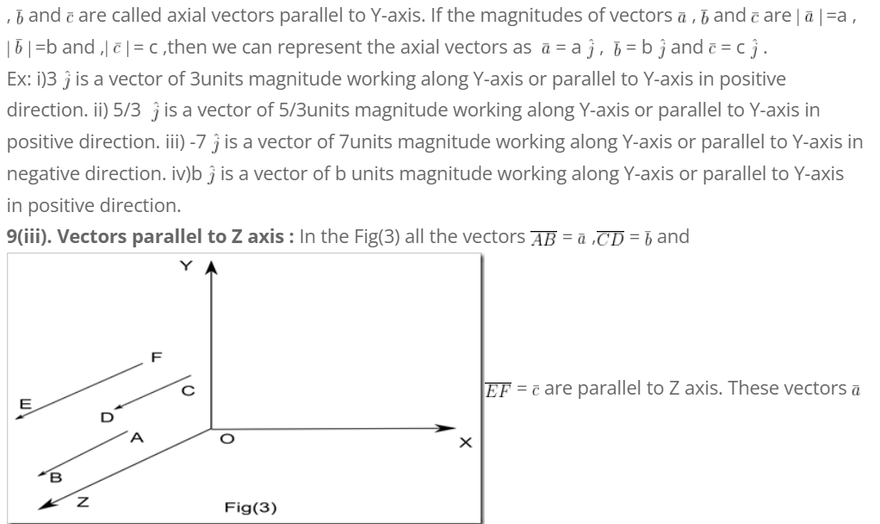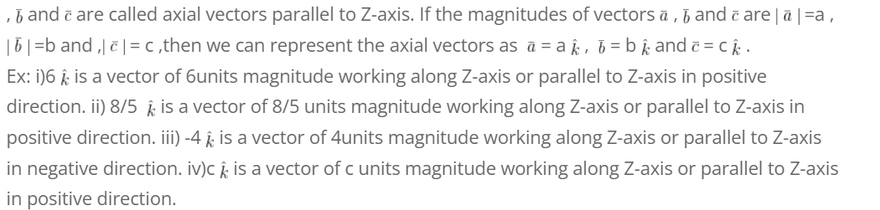10. Two dimensional vectors (or) Plane vectors: The vectors acting in XY-Plane or YZ-Plane or ZX-Plane are known as two dimensional vectors or also known as vectors in a plane.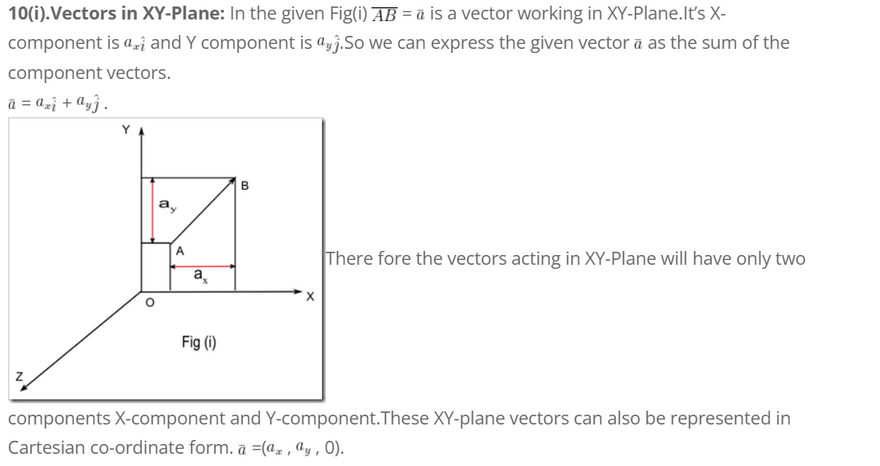Note: If X,Y co-ordinates exists(not equal to zero) and the Z co-ordinates does not exist(=0), such vectors will be in XY plane.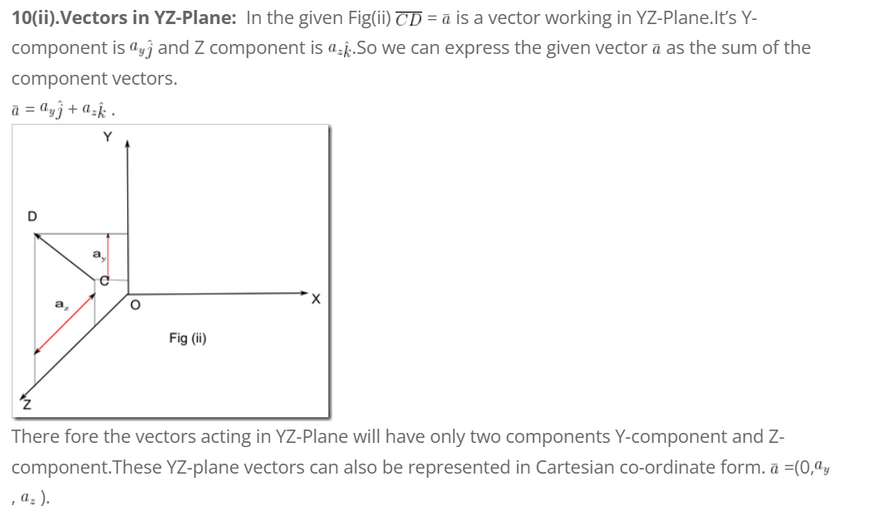Note: If Y,Z co-ordinates exists(not equal to zero) and the X co-ordinates does not exist(=0), such vectors will be in YZ plane.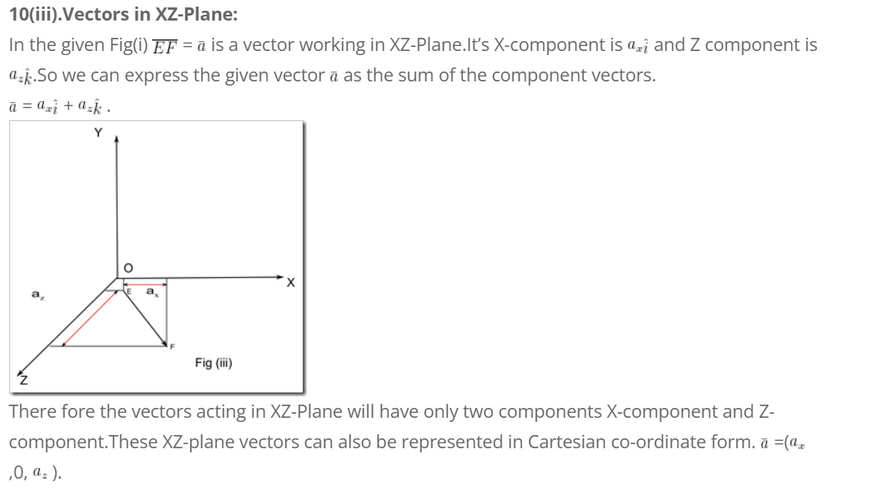Note: If X,Z co-ordinates exists(not equal to zero) and the Y co-ordinates does not exist(=0), such vectors will be in XZ plane.

11. Space Vector: If any vector possess components along all the three axes X,Y and Z such vectors are called space vectors.